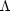Annu. Rev. Astron. Astrophys. 1984. 22: 157-184 Copyright © 1984 by Annual Reviews. All rights reserved

### 4. ALTERNATIVE PHYSICS

ENERGY VIOLATION     There are various proposals of alternative effective equations of state for matter, which result in violations of the energy condition (Equation 9b) through the existence of sufficiently large negative pressures. Thus, as well as transient solutions, one also obtains steady-state and periodic solutions (48); it is possible to avoid the initial singularity, e.g. by starting from a static initial situation that then becomes unstable or in a universe that goes from a collapse phase to a state of expansion (86). We discuss in turn several ways of generating negative pressures.

If the matter in the Universe has a bulk viscosity with the coefficient of bulk viscosity proportional to density, negative pressures arise such that energy violations sufficient to avoid an initial singularity occur (66, 113). However, it is not expected that this coefficient would have such a dependence on density in the case of ultrarelativistic fluids.

A classical conformal massive scalar field can generate violations of the energy conditions (45), and a massless conformal field coupled to pressureless matter can cause energy violations sufficient to cause a "bounce" instead of a singularity in a FLRW universe model (6). No such field is known to exist, but this situation may be regarded as analogous to the pion field, which mediates strong interactions in nuclear matter. The field would only be significant when very high densities had been attained, so the energy violation and bounce would occur for nuclear densities. Insofar as this is an adequate model of the fields dominant at these early times (which is unknown), the possibility arises of avoiding the singularity itself; indeed, if k = + 1 the universe model could "bounce" at each of a series of singularities, as envisaged in Tolman's oscillating universe (103).

Energy violation may be expected to arise when quantum effects are significant, for such violations may be regarded as the driving force for the Hawking radiation emitted by black holes (14, 44). For example, if one uses as the source of the gravitational field the expectation value of a quantum field theoretical energy-momentum tensor for a quantized scalar field possessing mass, coherence effects can give rise to negative pressures sufficiently large to violate the energy conditions of the singularity theorems. Then a Friedmann-like collapse can be arrested at elementary particle dimensions and changed to an expansion (74).

A particular case where energy violation is expected to arise is in the inflationary universe scenario (38, 53) of the GUTs, where a supercooled metastable state of unbroken symmetry (a "false vacuum") gives rise to an effective cosmological constantdominating other fields present. This in turn gives rise to an effective energy density µ' =/and effective pressure p' = -/; so (p + u)' = 0 (the limiting case of Equation 9a), which allows energy increase while conserving energy-momentum. (By Equation 5 the matter expands while its density stays constant, as in the steady-state universe, because of the negative pressures.) Now (µ + 3p)' = - 2/, so if> 0, then a violation of the energy condition (Equation 9b) must happen, causing an exponential expansion ("inflation"). This expansion solves the causal problems raised by horizons in the Universe because causally connected regions are now much larger than a Hubble radius (38, 53). While this energy violation cannot lead to avoidance of the initial singularity if thermal equilibrium is maintained (39), this condition is not necessarily fulfilled (79), and there is a possibility that the Universe could bounce and thereby avoid the initial singularity because of the energy violation (79). In the standard scenario, the GUTs phase is preceded by a radiation-dominated phase, with the usual singularity and horizons at early times; but one could conceive of the Universe as being created in the exponentially expanding state. Then its early properties will be those of the steady-state universe, without a curvature singularity or horizon.

Overall, it is clear that energy violation, in principle, could allow for avoidance of the initial singularity and permit a study of the Universe in its pre-expansion phase (101). When quantum effects dominate the equation of state, it is quite likely that such energy violation can occur.

OTHER EQUATIONS OF STATE     There are other proposals for alternative equations of state that do not violate the energy conditions but that disagree with the radiation form (Equation 4b); one still has an initial singularity, but it can be a cold or cool big bang, rather than a hot one (e.g. 40, 47, 84). The SHBB statements (A-C) and Equation 11 remain, except that the temperature T does not diverge; thus the basic view of the origin of the Universe remains, with only relatively small details being varied.